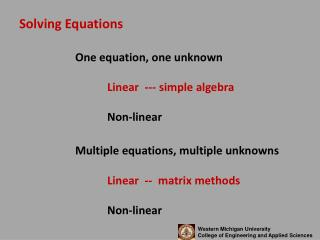DownloadDownload PresentationSolving Equations

# Solving Equations

Download Presentation## Solving Equations

- - - - - - - - - - - - - - - - - - - - - - - - - - - E N D - - - - - - - - - - - - - - - - - - - - - - - - - - -
##### Presentation Transcript

1. Solving Equations One equation, one unknown Linear --- simple algebra Non-linear Multiple equations, multiple unknowns Linear -- matrix methods Non-linear

2. Solving Equations Finding parameters to equations Linear --- Least Squares Non-linear Transform to linear form --- Least Squares Parameter Search

3. Solving Equations -- Non Linear One equation, one unknown X3 -17x+12 = 0 • Plot • Guess & Check • Successive Substitution • In Cell or Circular • Solver

4. Solving Equations -- Non Linear One equation, one unknown • Plot -- will always work if choose range properly • Guess & Check • Successive Substitution • In Cell or Circular May not always work. Can depend strongly on initial guess.

5. Solving Equations Multiple non-linear equations, multiple unknowns Finding parameters to equations Linear --- Least Squares Non-linear Transform to linear form --- Least Squares Parameter Search

6. Have data and think should fit a straight line: y = m *x + b

7. Minimize the square of the distance from the point to the line

8. Let (yi , xi) = ith data point pair Proposed line: y = m*x + b Then: ypi = m*xi + b and ei = yi - ypi Choose “m” and “b” to minimize: S(yi-ypi)2

9. Can show that the proper choice of “m” and “b” are:

10. One measure of how well the line fits the data is the Correlation Coefficient -- r In essence, the correlation coefficient explains how much of the variation of the data from the mean is explained by the line implies line has no predictive power implies line has perfect predictive power

11. Fitting Straight Lines to Data y = m*xn ln(y) = ln(m)+n*ln(x) plot ln(y) vs ln(x) (‘log - log’ plot) y= m*ebx ln(y) = ln(m) + b*x plot ln(y) vs x (‘semi-log’ plot)

12. What if you can’t transform the equation to a straight line form? Excel’s Solver to the rescue Rearrange equation so = 0 Guess the unknown parameters Evaluate the errors -- (need data) Adjust the parameters to minimize the sum of the squared error

13. Can apply this idea to fitting parameters to data much like least squares. • Guess the parameters. • Compute the “error” for each data point. • Let “solver” adjust the parameters to minimize the total squared error.